Functions and Algebra: Sketch and interpret functions and graphs

# Unit 3: Hyperbolic functions

Dylan Busa### Unit outcomes

By the end of this unit you will be able to:

• Identify the following characteristics of functions:
• continuous or discontinuous
• asymptotes.
• Sketch and find the equation of the graph $\scriptsize y=\displaystyle \frac{a}{x}+q$.
• Investigate and generalise the impact of $\scriptsize a$ and $\scriptsize q$ on $\scriptsize y=\displaystyle \frac{a}{x}+q$.

## What you should know

Before you start this unit, make sure you can

## Introduction

In Unit 1: Linear functions we learnt that functions are mathematical relations between two variables that map each element of the domain (the set of input values) to one and only one element in the range (the set of output values). We also saw that linear functions (functions of the form $\scriptsize y=ax+q$) produce straight line graphs.

In Unit 2: Quadratic functions we learnt that quadratic functions (functions of the form $\scriptsize y=ax^2+q$) produce parabolas when we sketch them. We learnt that parabolas are widely used in satellite dishes and mirrors in telescopes.

The hyperbolic function, that produces a graph we call a hyperbola, is also widely used in lenses and mirrors because it also focuses light to a single point. In this unit, we will explore the hyperbolic function in more detail and discover some very important properties that make it special.

# The hyperbolic function### Activity 3.1: Investigate the hyperbolic function

Time required: 30 minutes

What you need:

• a pen or pencil
• a calculator
• blank paper or a notebook

What to do:

Work through this scenario and answer the following questions:

A scientist was doing some experiments with helium gas. He measured the pressure a fixed amount of the gas was under (in $\scriptsize \text{kPa}$) for different volumes (in $\scriptsize \text{m}^3$) while making sure to keep the temperature of the gas constant at $\scriptsize 25~^\circ\text{C}$. Here are his results.

 Volume (in $\scriptsize \text{m}^3$) $\scriptsize 320$ $\scriptsize 300$ $\scriptsize 160$ $\scriptsize 150$ $\scriptsize 80$ $\scriptsize 64$ $\scriptsize 40$ Pressure (in $\scriptsize \text{kPa}$) $\scriptsize 50$ $\scriptsize 53.33$ $\scriptsize 100$ $\scriptsize 106.67$ $\scriptsize 200$ $\scriptsize 250$ $\scriptsize 400$
1. Plot these points on a Cartesian plane and join them with as smooth a curve as possible. Let volume be $\scriptsize x$ and pressure be $\scriptsize y$.
2. What happens to the pressure as the volume increases? Will the pressure ever reach zero?
3. What happens to the pressure as the volume decreases? Can the volume ever be zero?
4. Write a mathematical expression that relates the pressure and volume of this gas at this temperature. Here’s a hint: what is $\scriptsize 320\times50$ equal to?
5. Is the graph symmetrical? About what line do you think the graph is symmetrical?
6. Is there a piece of the graph that is missing? What happens if we have $\scriptsize x=-320$? Can you plot this missing piece of the graph?

What did you find?

1. If we plot these points on a Cartesian plane and then join them with a smooth curve, we get the graph in Figure 1.
2. As the volume increases (the $\scriptsize x$ values get bigger), the pressure decreases. It looks like eventually the pressure will be zero, but does this make any sense? Can the pressure of a gas ever be zero? No matter how big the volume gets, there will still be some pressure.
3. As the volume decreases (the $\scriptsize x$ values get smaller), the pressure increases. We know that physically the volume can never be zero. Everything in the universe always takes up some space.
4. $\scriptsize 320\times50=16~000$. But the interesting thing is that so does $\scriptsize 300\times53.33$ (if we round up), and $\scriptsize 160\times100$. In fact, if we multiple each $\scriptsize x$ and $\scriptsize y$ pair, the answer is always $\scriptsize 16~000$. This means that this graph is given by the expression $\scriptsize xy=16~000$.
5. It looks like the graph is symmetrical about the straight line that passes through the origin and has a gradient of 1. In other words, it looks like the graph is symmetrical about the line $\scriptsize y=x$ as shown in figure 2.Figure 2: Plot of volume vs pressure of a gas showing symmetry about the line $\scriptsize y=x$
6. We know that the equation of the graph is given by $\scriptsize xy=16~000$. Therefore, there is a piece of the graph missing because we know that $\scriptsize -320\times-50=16~000$. We could make each of the values we have negative and add these new points to complete the graph. The complete graph would look like Figure 3.Figure 3: Graph of the function $\scriptsize xy=16~000$

The hyperbola (the graph of the hyperbolic function) looks quite similar to a parabola. It almost looks like a parabola on its side. We can also see that the hyperbola, like the parabola, has an axis of symmetry. In Figure 3, this is the line $\scriptsize y=x$. But, can you see that there is another axis of symmetry – the line $\scriptsize y=-x$ (see Figure 4)?

We saw that the hyperbolic function is given by $\scriptsize xy=k$ where $\scriptsize k$ is some constant. We can rewrite this to look more like the other functions we have studied as $\scriptsize y=\displaystyle \frac{k}{x}$. This is called writing the equation in standard form. In the case above, the function relating the volume and pressure of the gas is $\scriptsize y=\displaystyle \frac{16~000}{x}$.

## The features of the hyperbolic function

Let’s do another activity to explore the features of the hyperbola in more detail.### Activity 3.2: Discover the properties of the hyperbolic function

Time required: 30 minutes

What you need:

• a pen or pencil
• a calculator
• blank paper or a notebook

What to do:

You are given the hyperbolic function $\scriptsize f(x)=\displaystyle \frac{3}{x}$.

1. Complete the following table of values for this function.
 $\scriptsize x$ $\scriptsize -3$ $\scriptsize -2$ $\scriptsize -1$ $\scriptsize -\displaystyle \frac{1}{3}$ $\scriptsize 0$ $\scriptsize \displaystyle \frac{1}{3}$ $\scriptsize 1$ $\scriptsize 2$ $\scriptsize 3$ $\scriptsize y$
2. Plot these points and join them with a smooth curve.
3. What happens when $\scriptsize x=0$?
4. What happens to $\scriptsize y$ when the value of $\scriptsize x$ gets very large?
5. What happens to $\scriptsize y$ when the value of $\scriptsize x$ gets very small?
6. Will the graph ever touch or cross either of the axes? Why or why not?
7. Is $\scriptsize y=\displaystyle \frac{3}{x}$ really a function? Test it and if so, write it in function notation.
8. What are the domain and range of this function?
9. About which two straight lines is the graph symmetrical?

What did you find?

1. The completed table of values.
 $\scriptsize x$ $\scriptsize -3$ $\scriptsize -2$ $\scriptsize -1$ $\scriptsize -\displaystyle \frac{1}{3}$ $\scriptsize 0$ $\scriptsize \displaystyle \frac{1}{3}$ $\scriptsize 1$ $\scriptsize 2$ $\scriptsize 3$ $\scriptsize y$ $\scriptsize -1$ $\scriptsize –\displaystyle \frac{3}{2}$ $\scriptsize -3$ $\scriptsize -9$ Undefined $\scriptsize 9$ $\scriptsize 3$ $\scriptsize \displaystyle \frac{3}{2}$ $\scriptsize 1$
2. If we plot the points and join them with a smooth curve, we get a hyperbola (Figure 5).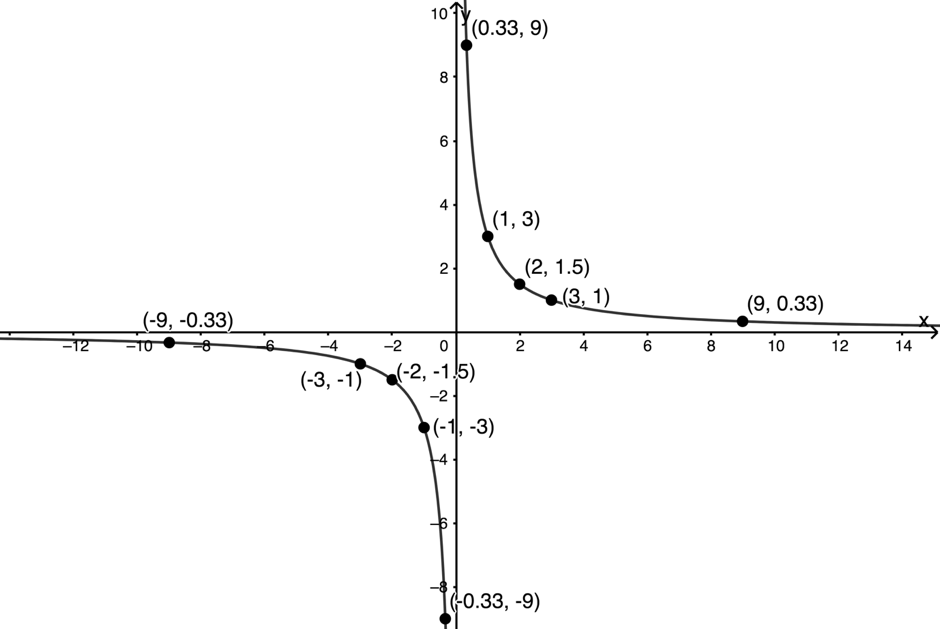Figure 5: Plot of $\scriptsize y=\displaystyle \frac{3}{x}$
3. When we let $\scriptsize x=0$, $\scriptsize \displaystyle \frac{3}{x}$ is undefined. We are never allowed to divide by zero. Therefore $\scriptsize x \neq0$.
4. As $\scriptsize x$ gets larger and larger, the value of $\scriptsize \displaystyle \frac{3}{x}$ gets smaller and smaller. We can see this in the graph. As the values of $\scriptsize x$ move off to the right or the left, the graph gets closer and closer to the x-axis.
5. As $\scriptsize x$ gets smaller and smaller, the value of $\scriptsize \displaystyle \frac{3}{x}$ gets bigger and bigger. We can see this in the graph. As the values of $\scriptsize x$ get closer to the y-axis, the graph increases or decreases rapidly.
6. We know that $\scriptsize x\neq0$. This means that the graph will never touch or cross the y-axis. We can rewrite the equation as $\scriptsize x=\displaystyle \frac{3}{y}$. Now we can also see that $\scriptsize y\neq0$. This means that the graph will never touch or cross the x-axis either.
7. If we use the vertical line test, we can see that at no point does any input value give more than one output value as shown in Figure 6. At $\scriptsize x=0$, however, the graph does not exist at all. We can rewrite it in function notation as $\scriptsize f(x)=\displaystyle \frac{3}{x}, x\neq 0$.
8. Domain: $\scriptsize {x:x\in \mathbb{R},x\neq0}$
Range: $\scriptsize {f(x):f(x)\in \mathbb{R},y\neq0}$
9. The graph is symmetrical about the lines $\scriptsize y=x$ and $\scriptsize y=-x$ (Figure 7).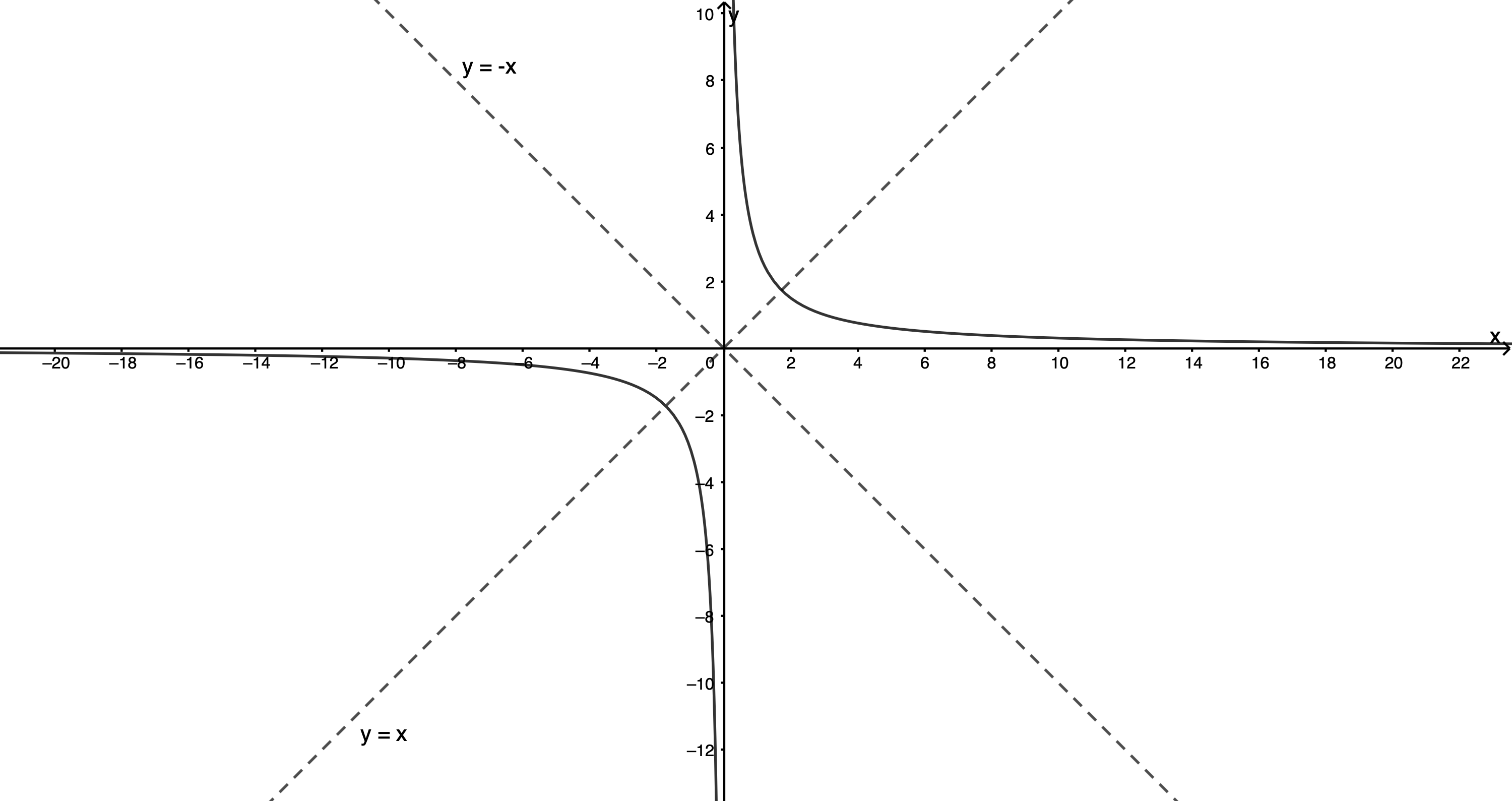Figure 7: Axes of symmetry of a hyperbola $\scriptsize y=\displaystyle \frac{3}{x}$

### Asymptotes and continuity

In Activity 3.2, we saw that for $\scriptsize y =\displaystyle \frac{3}{x}$, the value of $\scriptsize x$ can never be zero because we are never allowed to divide by zero. The graph gets closer and closer to the y-axis (where $\scriptsize x=0$) but never touches or crosses it. We call the y-axis an asymptote of the graph. An asymptote is a straight line that a curve gets closer and closer to without ever touching it.

But we also saw that we can rewrite the equation of the function as $\scriptsize x=\displaystyle \frac{3}{y}$. This shows us that $\scriptsize y\neq0$. Therefore, the graph gets closer and closer to the x-axis (where $\scriptsize y=0$) but never touches or crosses it. Therefore, the y-axis is also an asymptote of the graph.

We know that the hyperbola has two asymptotes. Look at the vertical asymptote in Figure 8, for example. The graph approaches the y-axis from the right. But to keep drawing the graph, we eventually have to pick up our pencil and start again on the left-hand side of the y-axis.

Because, we have to pick up our pencil to draw the whole graph, we say that the hyperbola is discontinuous. Look at the parabola and straight-line graphs in Figure 8. Do we ever need to pick up our pencil to draw these? Because we do not, we say that these functions are continuous.### Take note!

In general, hyperbolic functions of the form $\scriptsize f(x)=\displaystyle \frac{a}{x}$ have the following properties.

• The line $\scriptsize y=0$ (the x-axis) is a horizontal asymptote.
• The line $\scriptsize x=0$ (the y-axis) is a vertical asymptote.
• The function is discontinuous at the asymptotes.
• The domain of the function is $\scriptsize {x:x\in \mathbb{R},x\neq0}$.
• The range of the function is $\scriptsize {f(x):f(x)\in \mathbb{R},y\neq0}$.
• The line $\scriptsize y = x$ is an axis of symmetry.
• The line $\scriptsize y = -x$ is an axis of symmetry.

But what happens if we have the function $\scriptsize g(x)=\displaystyle \frac{3}{x}+1$? Now the function is of the form $\scriptsize y= \displaystyle \frac{a}{x}+q$. What does this graph look like?

## The effect of $\scriptsize \large q$ on the hyperbola of the form $\scriptsize \large y=\displaystyle \frac{a}{x}+q$?### Activity 3.3: Discover the effect of q on the hyperbola of the form y= a/x +q

Time required: 30 minutes

What you need:

• coloured pens or pencils
• a calculator
• blank paper or a notebook

What to do:

Part A

1. Plot the following hyperbolic functions on the same set of axes using a table of values.
1. $\scriptsize f(x)=\displaystyle \frac{3}{x}$
2.  $\scriptsize g(x)=\displaystyle \frac{3}{x}+1$
3.  $\scriptsize h(x)=\displaystyle \frac{3}{x}-2$
You should sketch each graph in a different colour.
2. What are the vertical asymptotes of $\scriptsize g(x)$ and $\scriptsize h(x)$? Are they the same as for $\scriptsize f(x)$?
3. What are the horizontal asymptotes of $\scriptsize g(x)$ and $\scriptsize h(x)$? Are they the same as for $\scriptsize f(x)$?
4. What is the domain and range of $\scriptsize g(x)$ and $\scriptsize h(x)$?
5. What are the axes of symmetry of $\scriptsize g(x)$ and $\scriptsize h(x)$?
6. What is the effect of the value of $\scriptsize q$ on the graph of $\scriptsize y=\displaystyle \frac{a}{x}+q$?

Part B

Now, if you have access to the internet, visit the hyperbolic function interactive simulation.Here you will find a hyperbolic function of the form $\scriptsize y=\displaystyle \frac{a}{x}+q$ with a slider to change the values of $\scriptsize q$.

1. Change the value of $\scriptsize q$ to confirm what you know about how the value of $\scriptsize q$ affects the hyperbola. How does this relate to how $\scriptsize q$ affects the straight line $\scriptsize y=ax+q$ and the parabola $\scriptsize y=ax^2+q$?
2. How does changing $\scriptsize q$ affect the vertical asymptote of the hyperbola?
3. How does changing $\scriptsize q$ affect the horizontal asymptote of the hyperbola?
4. How does changing $\scriptsize q$ affect the axes of symmetry of the hyperbola?

What did you find?

Part A

1. Here are plots of all three functions (Figure 9).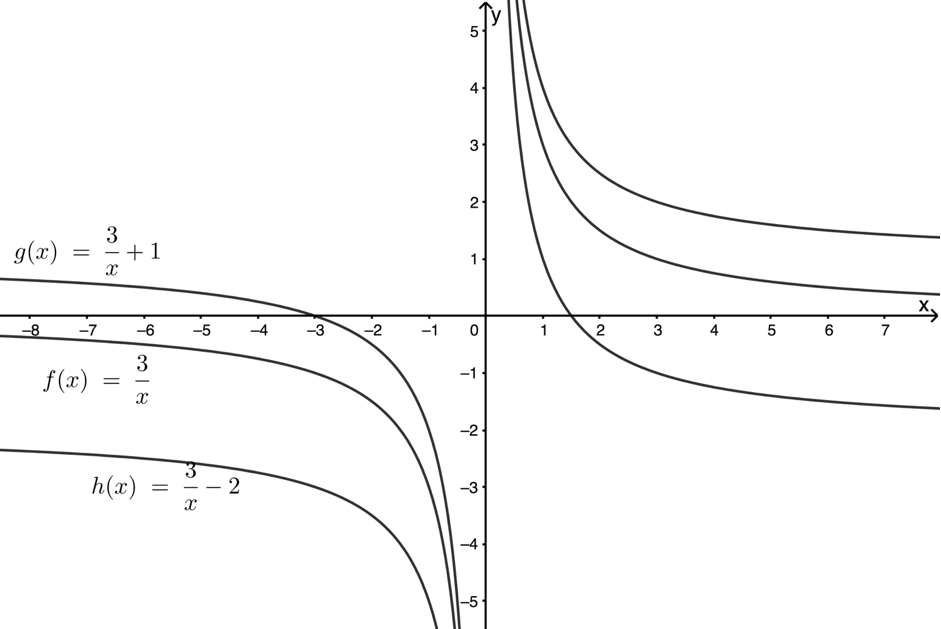Figure 9: Plots of $\scriptsize f(x)$, $\scriptsize g(x)$ and $\scriptsize h(x)$
2. The vertical asymptotes of $\scriptsize g(x)$ and $\scriptsize h(x)$ are both the y-axis or the line $\scriptsize x=0$. This is the same as $\scriptsize f(x)$.
3. The horizontal asymptote of $\scriptsize g(x)=\displaystyle \frac{3}{x}+1$ is the line $\scriptsize y=1$ (Figure 10).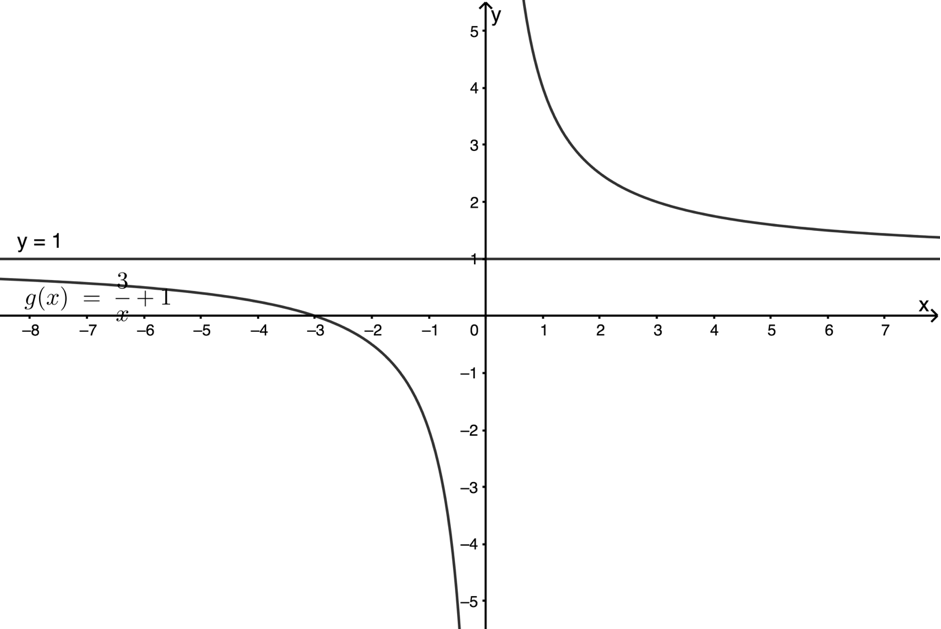Figure 10: Horizontal asymptote of $\scriptsize g(x)$

.
The horizontal asymptote of $\scriptsize h(x)=\displaystyle \frac{3}{x}-2$ is the line $\scriptsize y=-2$ (Figure 11).Figure 11: Horizontal asymptote of $\scriptsize h(x)$
4. The domain and range of $\scriptsize g(x)$ are:
Domain: $\scriptsize {x\ |\ x\in \mathbb{R},x\neq0}$
Range: $\scriptsize {f(x)\ |\ f(x)\in \mathbb{R},y\neq1}$
.
The domain and range of $\scriptsize h(x)$ are:
Domain: $\scriptsize {x\ |\ x\in \mathbb{R},x\neq0}$
Range: $\scriptsize {f(x)\ |\ f(x)\in \mathbb{R},y\neq-2}$
5. The axes of symmetry of $\scriptsize g(x)=\displaystyle \frac{3}{x}+1$ are the lines $\scriptsize y = x+1$ and $\scriptsize y = -x+1$ (Figure 12).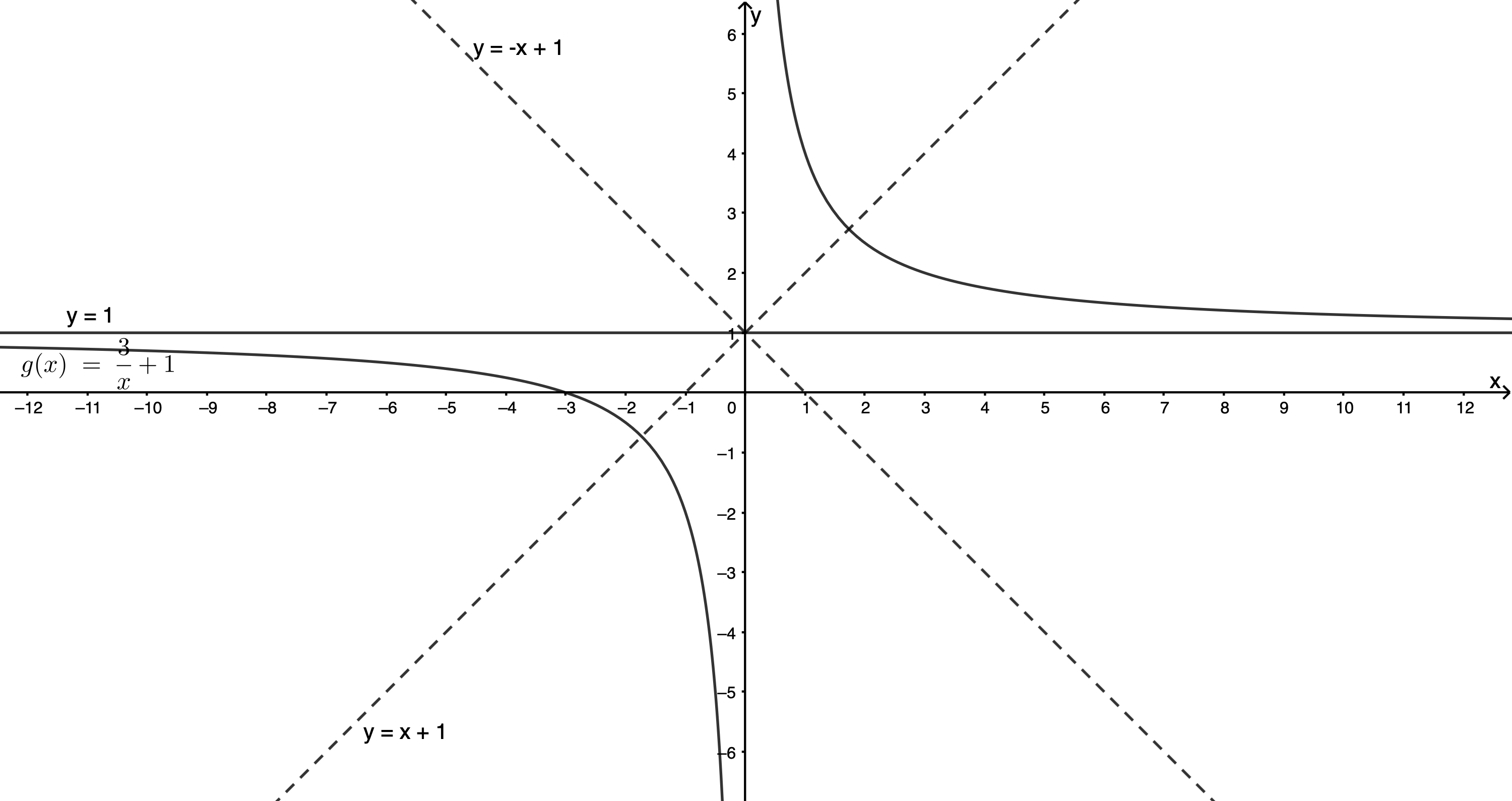Figure 12: Axes of symmetry of $\scriptsize g(x)$

.
The axes of symmetry of $\scriptsize h(x)=\displaystyle \frac{3}{x}-2$ are the lines $\scriptsize y = x-2$ and $\scriptsize y = -x-2$ (Figure 13).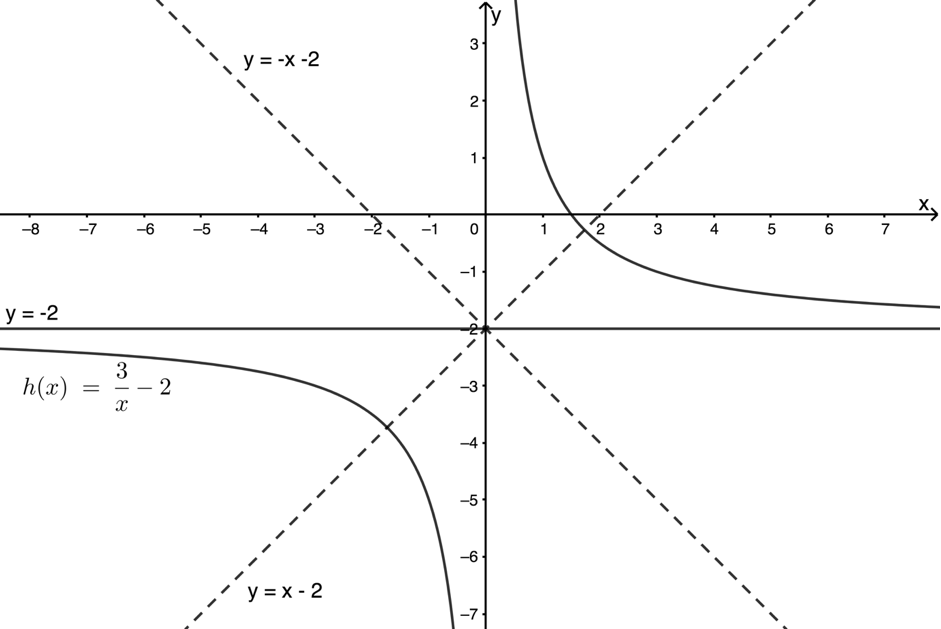Figure 13: Axes of symmetry of $\scriptsize h(x)$
6. The effect of $\scriptsize q$ on the graph of $\scriptsize y=\displaystyle \frac{a}{x}+q$ is to move the graph vertically up or down. This moves the graph itself up or down as well as moving the horizontal asymptote and lines of symmetry up or down as well.

Part B

1. As we increase the value of $\scriptsize q$, the hyperbola shifts up vertically. Decreasing the value of $\scriptsize q$ shifts the graph down. This is exactly the same affect that changing the value of $\scriptsize q$ has on the straight line $\scriptsize y=ax+q$ and the parabola $\scriptsize y=ax^2+q$.
2. Changing the value of $\scriptsize q$ has no effect on the vertical asymptote. The graph does not shift left or right at all.
3. As changing $\scriptsize q$ shifts the hyperbola up and down, it also shifts the hyperbola’s horizontal axis up or down by the same amount.
4. In the same way as changing $\scriptsize q$ shifts the graph and the horizontal asymptote up or down, it also shifts the axes of symmetry up or down by the same amount.

We have discovered that the effect of $\scriptsize q$ in $\scriptsize y=\displaystyle \frac{a}{x}+q$ is the same as in $\scriptsize y=ax+q$ and $\scriptsize y=ax^2+q$. Changing $\scriptsize q$ shifts the graph vertically up or down. The horizontal asymptote and axes of symmetry are also shifted up and down by the same amount.

## Sketching hyperbolic functions of the form $\scriptsize \large y=\displaystyle \frac{a}{x}+q$

Have a look at this next example.### Example 3.1

Sketch the following hyperbolic functions by first finding their asymptotes and axes of symmetry and then by finding any two other points on the graph. These can include any intercepts with the axes if they exist.

1. $\scriptsize f(x)=\displaystyle \frac{1}{x}-1$
2. $\scriptsize j(x)=\displaystyle \frac{3}{x}-\displaystyle \frac{3}{2}$

Solutions

1. $\scriptsize f(x)=\displaystyle \frac{1}{x}-1$ is of the form $\scriptsize y=\displaystyle \frac{a}{x}+q$ but has been shifted one unit down. Therefore, the graph’s horizontal asymptote is the line $\scriptsize y=-1$. The axes of symmetry are the lines $\scriptsize y=x-1$ and $\scriptsize y=-x-1$.
.
We can find the x-intercept if we make $\scriptsize y=0$.
\scriptsize \begin{align*} 0&=\displaystyle \frac{1}{x}-1\\ \therefore \displaystyle \frac{1}{x}&=1\\ \therefore x&=1 \end{align*}
.
We can find another point by substituting $\scriptsize x=2$ into the function. Therefore $\scriptsize f(2)=\displaystyle \frac{1}{2}-1=-\displaystyle \frac{1}{2}$.
.
Here is the sketch of $\scriptsize f(x)$ (Figure 14).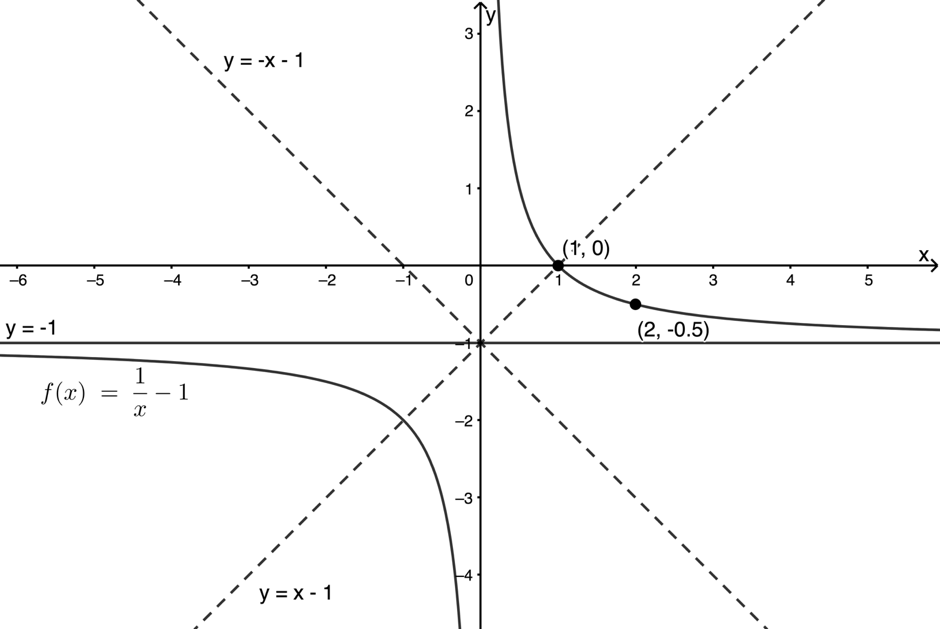Figure 14: Sketch of $\scriptsize f(x)$
2. $\scriptsize j(x)=\displaystyle \frac{3}{x}-\displaystyle \frac{3}{2}$ is of the form $\scriptsize y=\displaystyle \frac{a}{x}+q$ but has been shifted down by one and a half units. Therefore, the graph’s horizontal asymptote is the line $\scriptsize y=-\displaystyle \frac{3}{2}$. The axes of symmetry are the lines $\scriptsize y=x-\displaystyle \frac{3}{2}$ and $\scriptsize y=-x-\displaystyle \frac{3}{2}$.
.
We can find the x-intercept if we make $\scriptsize y=0$.
\scriptsize \begin{align*} 0&=\displaystyle \frac{3}{x}-\displaystyle \frac{3}{2}\\ \therefore \displaystyle \frac{3}{x}&=\displaystyle \frac{3}{2}\\ \therefore x&=2 \end{align*}
.
We can find another point by substituting $\scriptsize x=1$ into the function. Therefore $\scriptsize j(1)=\displaystyle \frac{3}{1}-\displaystyle \frac{3}{2}=\displaystyle \frac{3}{2}$.
.
Here is the sketch of $\scriptsize j(x)$ (Figure 15).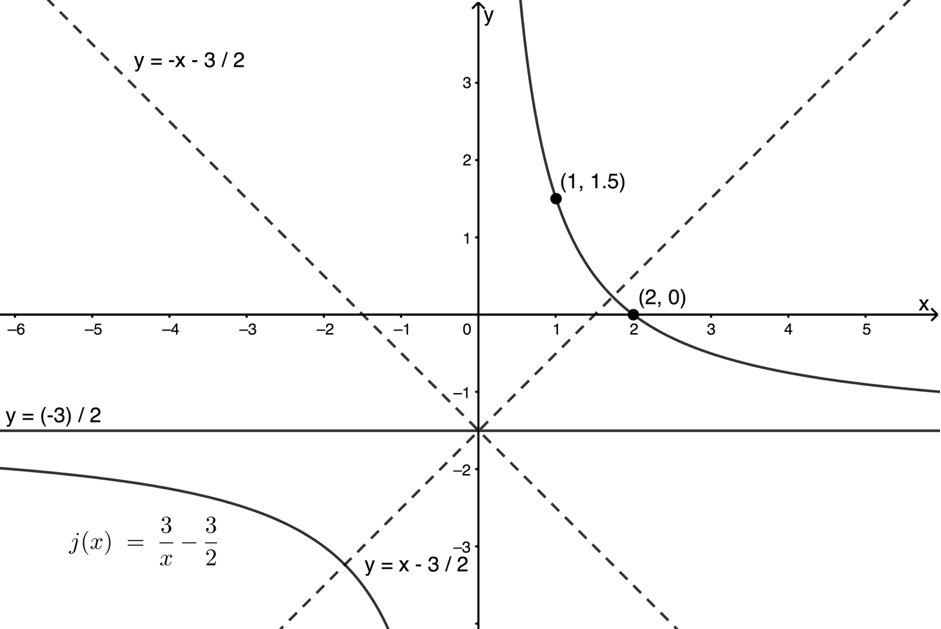Figure 15: Sketch of $\scriptsize j(x)$

## The effect of $\scriptsize \large a$ on $\scriptsize \large y=\displaystyle \frac{a}{x}+q$

So far, we have discovered what effect $\scriptsize q$ has on the hyperbolic function of the form $\scriptsize y=\displaystyle \frac{a}{x}+q$. But what is the effect of $\scriptsize a$? Work through this next example to find out.### Example 3.2

1. Sketch the following hyperbolic functions on the same set of axes by first finding their asymptotes and axes of symmetry and then by finding any two other points on the graph. These can include any intercepts with the axes if they exist.
$\scriptsize f(x)=\displaystyle \frac{1}{x}+1$
$\scriptsize g(x)=\displaystyle \frac{3}{x}+1$
$\scriptsize h(x)=-\displaystyle \frac{2}{x}+1$
2. What affect does the value of $\scriptsize a$ have on the shape and position of the hyperbolic function of the form $\scriptsize y=\displaystyle \frac{a}{x}+q$?

Solutions

1. All three functions have a value of $\scriptsize q=1$. Therefore, all the functions will have the same horizontal asymptote and the same axes of symmetry. We just need to find two additional points on each graph to help us sketch them.
.
First, we will find the x-intercepts.
\scriptsize \begin{align*} f(x)=0:\\ \therefore \displaystyle \frac{1}{x}+1&=0\\ \therefore \displaystyle \frac{1}{x}&=-1\\ \therefore x&=-1\\ g(x)=0:\\ \therefore \displaystyle \frac{3}{x}+1&=0\\ \therefore \displaystyle \frac{3}{x}&=-1\\ \therefore x&=-3\\ h(x)=0:\\ \therefore -\displaystyle \frac{2}{x}+1&=0\\ \therefore \displaystyle \frac{2}{x}&=1\\ \therefore x&=2\\ \end{align*}
.
Now we can find any other point on each graph. We will choose convenient values of $\scriptsize x$ to work with for each function.
\scriptsize \begin{align*} f(-2)=\displaystyle \frac{1}{(-2)}+1=\displaystyle \frac{1}{2}\\ g(-1)=\displaystyle \frac{3}{(-1)}+1=-2\\ h(1)=-\displaystyle \frac{2}{1}+1=3\\ \end{align*}
.
With this information, we can plot each of the functions (Figure 16).Figure 16: Sketches of $\scriptsize f(x)$, $\scriptsize g(x)$ and $\scriptsize h(x)$
2. From the graphs we plotted, we can see that the bigger the of $\scriptsize a$, the wider the hyperbola is. Look at the difference of the shape of $\scriptsize f(x)=\displaystyle \frac{1}{x}+1$ and $\scriptsize g(x)=\displaystyle \frac{3}{x}+1$ in Figure 16.
.
Also, the sign of $\scriptsize a$ determines where the graph is plotted. If $\scriptsize a \gt 0$, the graph is in the first and third quadrants of the Cartesian plane (see Figure 16). If $\scriptsize a \lt 0$, the graph is in the second and fourth quarters of the Cartesian plane (see Figure 16).

### Note

We call each quarter of the Cartesian plane a quadrant and we number the quadrants from the top right in an anti-clockwise direction.Here you will find a hyperbolic function of the form $\scriptsize y=\displaystyle \frac{a}{x}+q$ with sliders to change the values of $\scriptsize a$ and $\scriptsize q$. Spend some time playing with it to make sure that you understand how changing the values of $\scriptsize a$ and $\scriptsize q$ affects the shape and location of the hyperbola of the form $\scriptsize y=\displaystyle \frac{a}{x}+q$.### Example 3.3

Sketch the function $\scriptsize q(x)=-\displaystyle \frac{3}{4x}+2$ and label the intercepts, asymptotes and axes of symmetry.

Solution

First, we need to write the function in the form $\scriptsize y=\displaystyle \frac{a}{x}+q$. Therefore $\scriptsize q(x)=-\displaystyle \frac\frac{3}{4}}{x}+$, $\scriptsize a=-\displaystyle \frac{3}{4}$ and $\scriptsize q=2$.

Because $\scriptsize a \lt 0$, we know that the graph is plotted in quadrants 2 and 4. We also know that the horizontal asymptote is the line $\scriptsize y=2$ and that the axes of symmetry are the lines $\scriptsize y=x+2$ and $\scriptsize y=-x+2$.

Because the x-axis is a vertical asymptote, we know there are no y-intercepts but we can find the x-intercepts by letting $\scriptsize y=0$.

\scriptsize \begin{align*} f(x)=-\displaystyle \frac\frac{3}{4} }{x}+2&=0\\ \therefore -\displaystyle \frac\frac{3}{4}}{x}&=-2\\ \therefore -\displaystyle \frac{3}{4}&=-2x &&\text{We multiplied both side of the equation by } x\\ \therefore x&=\displaystyle \frac{3}{8} \end{align*

Finally, we can sketch the graph as shown in Figure 17.Figure 17: Sketch of $\scriptsize q(x)=-\displaystyle \frac{3}{4x}+2$### Exercise 3.1

1. Sketch the function $\scriptsize g(x)=\displaystyle \frac{2}{x}+4$ and label the intercepts, asymptotes and axes of symmetry.
2. Sketch the function $\scriptsize r(x)+6=-\displaystyle \frac{4}{x}$ and label the intercepts, asymptotes and axes of symmetry.

The full solutions are at the end of the unit.### Take note!

Figure 18 shows a summary of what we know about the hyperbolic function of the form $\scriptsize y=\displaystyle \frac{a}{x}+q$.Figure 18: Summary of the hyperbolic function of the form $\scriptsize y=\displaystyle \frac{a}{x}+q$

In general, hyperbolic functions of the form $\scriptsize f(x)=\displaystyle \frac{a}{x}+q$ have the following properties:

• The line $\scriptsize y=q$ is a horizontal asymptote.
• The line $\scriptsize x=0$ (the y-axis) is a vertical asymptote.
• The function is discontinuous at the asymptotes.
• The domain of the function is $\scriptsize \{x\ |\ x\in \mathbb{R},x\neq0\}$.
• The range of the function is $\scriptsize \{f(x)\ |\ f(x)\in \mathbb{R},y\neq q\}$.
• The line $\scriptsize y = x+q$ is an axis of symmetry.
• The line $\scriptsize y = -x+q$ is an axis of symmetry.

# Find the equation of a hyperbolic function

So far, we know how to sketch the graph of hyperbolic functions of the form $\scriptsize y=\displaystyle \frac{a}{x}+q$. Have a look at the next example to see how to find the equation of the function from the graph.### Example 3.4

The graph below is of a hyperbola of the form $\scriptsize y= \displaystyle \frac{a}{x} +q$. $\scriptsize \text{A}$ is the point $\scriptsize (-2,4)$ and the horizontal asymptote is the line $\scriptsize y=2$. Find the equation of the function.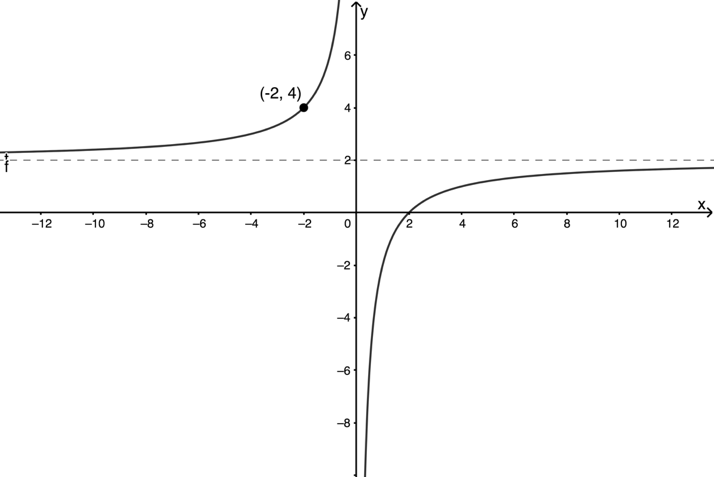Solution

The horizontal asymptote is $\scriptsize y=2$. Therefore, we know that $\scriptsize q=2$ and hence that $\scriptsize q(x)=\displaystyle \frac{a}{x}+2$.

The graph is in the 2nd and 4th quadrants. Therefore, we know that $\scriptsize a\lt0$

The point $\scriptsize (-2,4)$ lies on the graph so we can substitute these values into the equation to solve for $\scriptsize a$.
.
\scriptsize \begin{align*} f(-2)&=4\\ \therefore 4&=\displaystyle \frac{a}{-2}+2\\ \therefore \displaystyle \frac{a}{-2}&=2\\ \therefore a&=-4 \end{align*}
.
$\scriptsize q(x)=-\displaystyle \frac{4}{x}+2$### Exercise 3.2

1. A hyperbola of the form $\scriptsize \displaystyle \frac{a}{x}+q$ has a horizontal asymptote of $\scriptsize y=-\displaystyle \frac{2}{3}$ and an x-intercept of $\scriptsize (-\displaystyle \frac{5}{4},0)$. What is the equation of the function?
2. Given the following graph, match each function with the following equations:
1. $\scriptsize y=\displaystyle \frac{3}{2x}$
2. $\scriptsize y=\displaystyle \frac{3}{7x}$
3. $\scriptsize y=\displaystyle \frac{2}{x}$
4. $\scriptsize y=-\displaystyle \frac{4}{x}$
5. $\scriptsize y=-\displaystyle \frac{5}{2x}$
.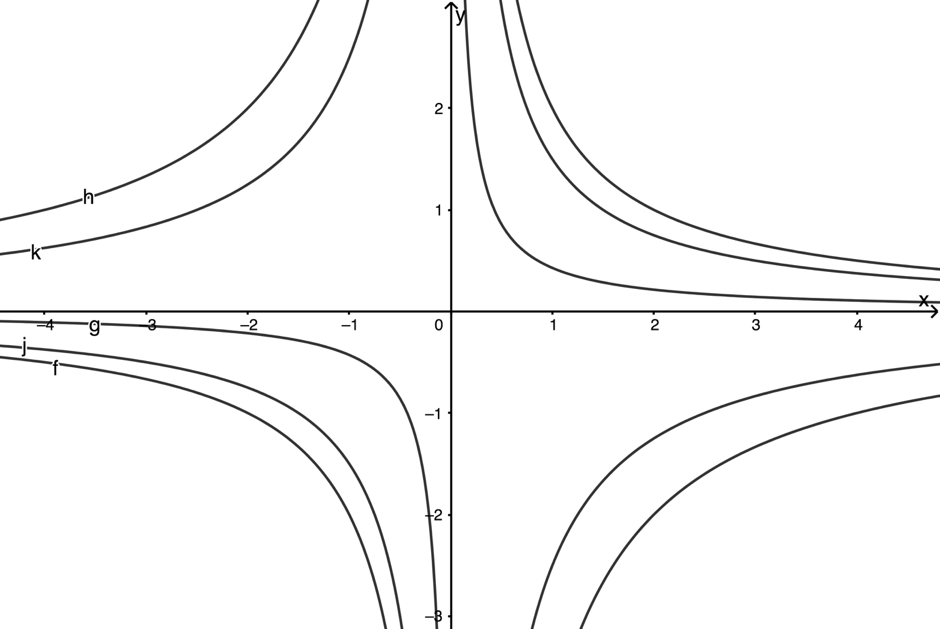3. A hyperbola has an equation of $\scriptsize 2x(y-2)=5$. State:
1. the equations of the asymptotes
2. the equations of the axes of symmetry
3. the domain
4. the range.

The full solutions are at the end of the unit.

## Summary

In this unit you have learnt the following:

• How to sketch hyperbolic functions of the form $\scriptsize y=\displaystyle \frac{a}{x}+q$.
• How to find the equation of graphs of the form $\scriptsize y=\displaystyle \frac{a}{x}+q$.
• The effect of $\scriptsize a$ and $\scriptsize q$ on the shape and position of $\scriptsize y=\displaystyle \frac{a}{x}+q$.
• What an asymptote is and how to find the asymptotes of a hyperbola of the form $\scriptsize y=\displaystyle \frac{a}{x}+q$.
• What is meant by a continuous and a discontinuous function.

# Unit 3: Assessment

#### Suggested time to complete: 40 minutes

1. Given $\scriptsize y=-\displaystyle \frac{3}{x}+2$, determine the intercepts with the axes.
2. Given the function $\scriptsize xy=-4$.
1. Sketch the function.
2. Give the equations of the asymptotes.
3. Looking at the graph, what are the coordinates of the point on the graph symmetrical to $\scriptsize (1,4)$ about the line $\scriptsize y=x$?
4. Does the point $\scriptsize (\displaystyle \frac{1}{2},-8)$ lie on the graph?
3. A hyperbola has an equation of $\scriptsize 3x(y+3)=6$.
1. State the equations of the asymptotes.
2. State the equations of the axes of symmetry.
3. State the domain.
4. State the range.
5. Sketch the function.
4. Without sketching the graphs, describe in words the differences between the following functions:
1. $\scriptsize f(x)=\displaystyle \frac{2}{x}$ and $\scriptsize g(x)=\displaystyle \frac{2}{x}+2$
2. $\scriptsize s(x)=\displaystyle \frac{2}{x}$ and $\scriptsize t(x)=-\displaystyle \frac{2}{x}$
3. $\scriptsize a(x)=\displaystyle \frac{2}{x}$ and $\scriptsize b(x)=-\displaystyle \frac{2}{x}+2$
4. $\scriptsize q(x)=\displaystyle \frac{2}{x}$ and $\scriptsize r(x)=\displaystyle \frac{4}{x}$
5. What is the equation of the hyperbolic function of the form $\scriptsize y=\displaystyle \frac{a}{x}+q$ which has an axis of symmetry of $\scriptsize y=x-2$ and an x-intercept of $\scriptsize (\displaystyle \frac{3}{2},0)$?
6. Look at the sketched functions $\scriptsize f(x)=2x^2-4$ and $\scriptsize g(x)=-\displaystyle \frac{2}{x}-4$.
.1. At what point(s) do the graphs intersect (or cross each other)?
2. For which values of $\scriptsize x$ is $\scriptsize g(x)\gt f(x)$. In other words, for which values of $\scriptsize x$ is the graph of $\scriptsize g(x)$ above the graph of $\scriptsize f(x)$?

The full solutions are at the end of the unit.

# Unit 3: Solutions

### Exercise 3.1

1. .2. .Back to Exercise 3.1

### Exercise 3.2

1. The horizontal asymptote is $\scriptsize y=-\displaystyle \frac{2}{3}$. Therefore $\scriptsize q=-\displaystyle \frac{2}{3}$. Therefore the equation is $\scriptsize y=\displaystyle \frac{a}{x}-\displaystyle \frac{2}{3}$.
But the x-intercept is $\scriptsize (-\displaystyle \frac{5}{4},0)$. Substitute this point into the equation and solve for $\scriptsize a$.
.
\scriptsize \begin{align*} y&=\displaystyle \frac{a}{x}-\displaystyle \frac{2}{3}\\ \therefore 0&=\displaystyle \frac{a}{-\displaystyle \frac{5}{4}}-\displaystyle \frac{2}{3}\\ \therefore \displaystyle \frac{a}\frac{5}{4}}&=-\displaystyle \frac{2}{3}\\ \therefore a&=\displaystyle \frac{2}{3} \times \displaystyle \frac{4}{5}=-\displaystyle \frac{8}{15} &&\text{Multiply through by the inverse of the denominator} \end{align*
.
The equation is $\scriptsize y=-\displaystyle \frac{8}{15x}-\displaystyle \frac{2}{3}$.
2. .
1. $\scriptsize j(x)=\displaystyle \frac{3}{2x}$
2. $\scriptsize g(x)=\displaystyle \frac{3}{7x}$
3. $\scriptsize f(x)=\displaystyle \frac{2}{x}$
4. $\scriptsize h(x)=-\displaystyle \frac{4}{x}$
5. $\scriptsize k(x)=-\displaystyle \frac{5}{2x}$
3. .
\scriptsize \begin{align} 2x(y-2) & =5 \\ \therefore y-2 & =\displaystyle \frac{5}{2x} \\ \therefore y & =\displaystyle \frac{5}{2x}+2 \end{align}
1. Horizontal asymptote:
$\scriptsize y=2$
Vertical asymptote:
$\scriptsize x=0$
2. Axes of symmetry:
$\scriptsize y=x+2$ and $\scriptsize y=-x+2$
3. Domain: $\scriptsize \{x\ |\ x\in \mathbb{R},x\ne 0\}$
4. Range: $\scriptsize \{y\ |\ y\in \mathbb{R},y\ne 2\}$

Back to Exercise 3.2

### Unit 3: Assessment

1. $\scriptsize y=-\displaystyle \frac{3}{x}+2$
x-intercept (let $\scriptsize y=0$):
\scriptsize \begin{align*} -\displaystyle \frac{3}{x}+2&=0\\ \therefore \displaystyle \frac{3}{x}&=2\\ \therefore 2x&=3\\ \therefore x&=\displaystyle \frac{3}{2}\\ \end{align*}
$\scriptsize \therefore$ x-intercept is the point  $\scriptsize (\displaystyle \frac{3}{2},0)$
2. .
1. $\scriptsize xy=-4$. Therefore, $\scriptsize y=-\displaystyle \frac{4}{x}$. $\scriptsize q=0$ so the horizontal asymptote is $\scriptsize y=0$ and the graph passes through the points $\scriptsize (-2,-2)$ and $\scriptsize (2,2)$.
.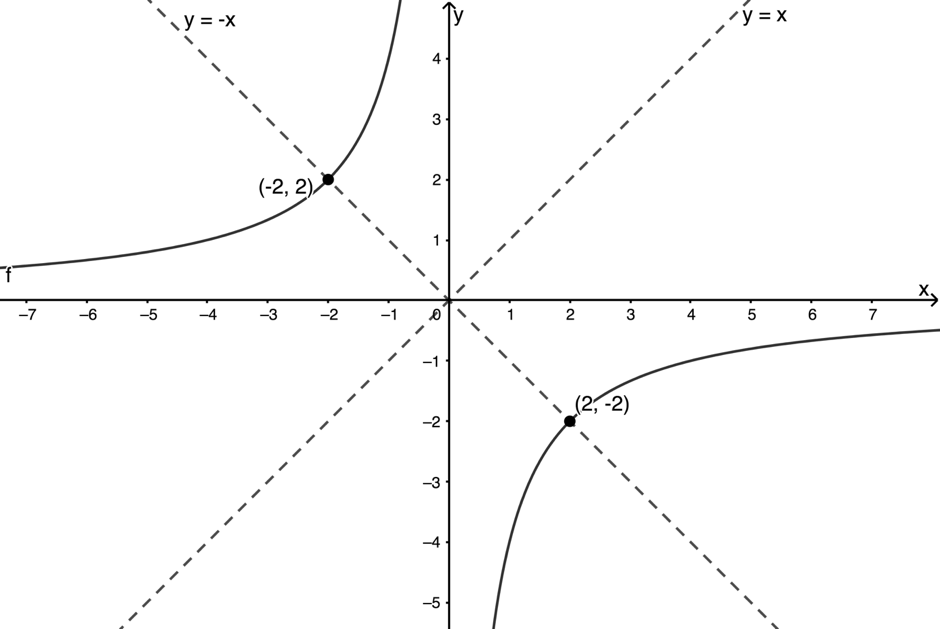2. The horizontal asymptote is $\scriptsize y=0$.
The vertical asymptote is $\scriptsize x=0$.
3. The point $\scriptsize (4,1)$ is symmetrical to the point $\scriptsize (1,4)$ about the line $\scriptsize y=x$.
.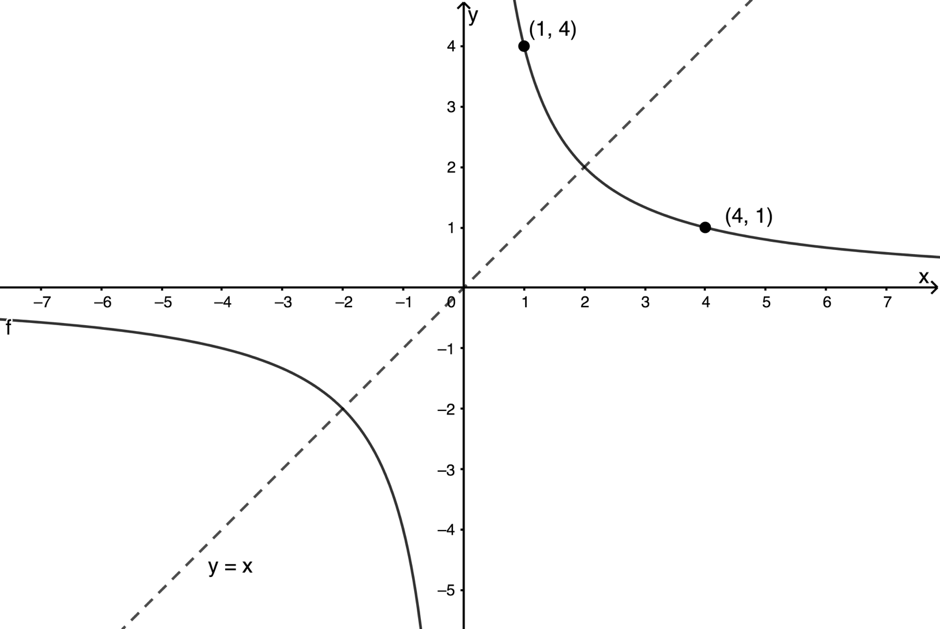4. We know the equation is $\scriptsize xy=4$. However $\scriptsize \displaystyle \frac{1}{2}\times-8\neq4$. Therefore the point does not lie on the graph.
3. .
1. .
$\scriptsize 3x(y+3)=6$. Write the equation in standard form.
\scriptsize \begin{align*} 3x(y-3)&=6\\ \therefore y+3&=\displaystyle \frac{6}{3x}\\ \therefore y&=\displaystyle \frac{2}{x}-3 \end{align*}
The vertical asymptote is $\scriptsize x=0$.
The horizontal asymptote is $\scriptsize y=-3$.
2. The axes of symmetry are $\scriptsize y=x-3$ and $\scriptsize y=-x-3$.
3. Domain: $\scriptsize \{x\ |\ x\in \mathbb{R},x\neq0\}$
4. Range: $\scriptsize \{f(x)\ |\ f(x)\in \mathbb{R},f(x)\neq -3$
5. .4. .
1. $\scriptsize g(x)$ is the same shape as $\scriptsize f(x)$ but has been shifted $\scriptsize 2$ units up.
2. $\scriptsize t(x)$ is the same shape as $\scriptsize s(x)$ but exists in quadrants 2 and 4 rather than quadrants 1 and 3.
3. $\scriptsize b(x)$ is the same shape as $\scriptsize a(x)$ but has been shifted $\scriptsize 2$ units up and exists in quadrants 2 and 4 rather than quadrants 1 and 3.
4. $\scriptsize r(x)$ is a narrower graph than $\scriptsize q(x)$
5. The axis of symmetry is the line $\scriptsize y=x-2$. Therefore, $\scriptsize q=-2$. So, the equation is $\scriptsize y=\displaystyle \frac{a}{x}-2$.
.
But $\scriptsize (\displaystyle \frac{3}{2},0)$ is the x-intercept. Substitute this point into the equation and solve for $\scriptsize a$.
\scriptsize \begin{align*} y=\displaystyle \frac{a}{x}-2\\ \therefore 0=\displaystyle \frac{a}\frac{3}{2}}-2\\ \therefore 2=\displaystyle \frac{a}\frac{3}{2}}\\ \therefore \displaystyle \frac{4}{3}=a &&\text{Multiply through by the inverse of the denominator} \end{align*
.
The equation is $\scriptsize y=\displaystyle \frac{4}{3x}-2$.
6. .
1. $\scriptsize f(x)=2x^2-4$ and $\scriptsize g(x)=\displaystyle \frac{2}{x}-4$. The graphs intersect when $\scriptsize f(x)= g(x)$.
.
\scriptsize \begin{align*} f(x)&=g(x)\\ \therefore 2x^2-4&=\displaystyle \frac{2}{x}-4\\ \therefore 2x^2&=\displaystyle \frac{2}{x}\\ \therefore 2x^3&=2\\ \therefore x^3&=1 &&\text{take the cube root of both sides of the equation}\\ \therefore x&=1 &&\text{The only number which multiplied by itself three times gives }1 \text{ is }1 \end{align*}
.
Now we can substitute $\scriptsize x=1$ into either function. $\scriptsize g(1)=\displaystyle \frac{2}{1}-4=-2$. The graphs intersect at the point $\scriptsize (1,-2)$.
2. By looking at the graph, we can see that $\scriptsize g(x) \gt f(x)$ when $\scriptsize 0 \lt x \lt 1$.
.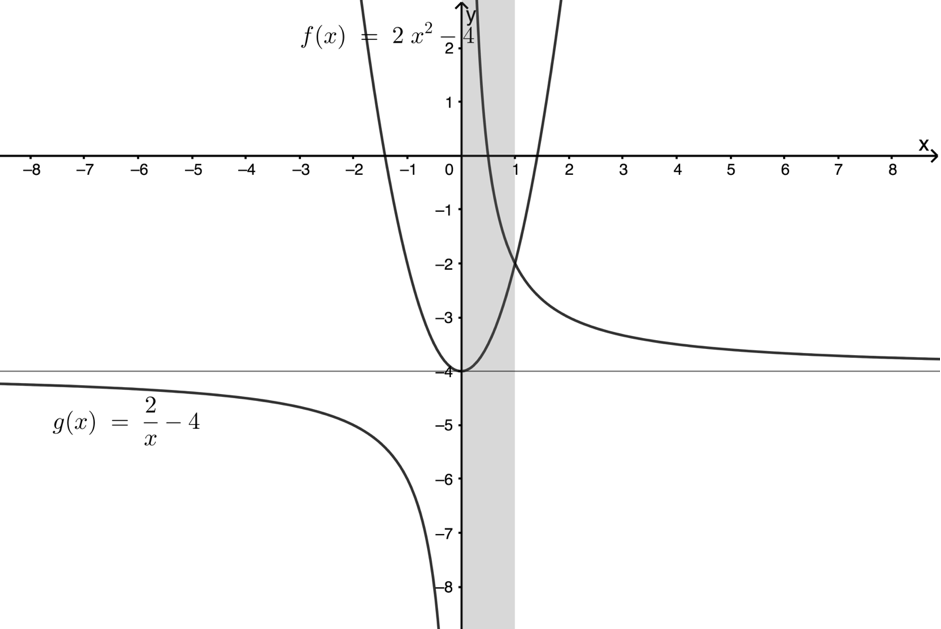Back to Unit 3: Assessment